Physics Paper 2, May/June. 2012
 Questions: 1 2 3 4 5 6 7 8 9 10 11 12 13 14 15 Main
Weakness/Remedies
Strength

Question 12

(a) (i) What is a machine?
(ii) State two uses of gears
(iii) Define the velocity ratio for a pair of gear system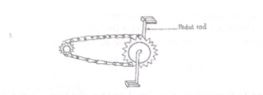The diagram above illustrates the gears system of a bicycle

1. Determine its velocity ratio
2. If the bicycle has an efficiency of 90%, calculate the effort required to overcome a load of 70 N.
3. Why is the calculated effort less than the actual effort required?
_____________________________________________________________________________________________________

This question was very popular among the candidates.
Part (a)(i) majority of the responding candidates got the definition correct. Few candidates however, stated the additional information that ought's to be added to the actual as their main definition of machine.
Part a(ii) uses of gears was correctly stated by few candidates. Few candidates wrote where gears are used (e.g. in bicycles, motor cars, pulley systems e.t.c) and not the demand of the question.
Part (a)(iii) many candidates wrote
V. R = Number of teeth in the driving wheel Number of teeth in the driven wheel
Which was wrong.

Part (a(iv) was poorly attempted as most candidates failed to observe the constructional input in a gear that can achieve the desired goal.
Part (b)(i) to (iii). The problem most candidates had was on how to obtain the velocity ratio. Many of them did not realize that they need to (oc) identify and ((S) physically count the number of teeth on tho driven and diivmg wheels as illustrated in the diagram in the question, Those who succeeded in deriving the velocity ratio had no difficulty in solving the remaining parts of the questions.
Performance was average

1. A machine is a device in which effort applied at one end is used to overcome a greater load/resistance at another point.

It makes work easier

1. Uses of gears

e.g - to change direction of motion

1. to change speed
2. to magnify force

(iii)
Velocity ratio
Velocity ratio is defined as ratio of number of teeth on driven wheel to number of teeth on driving wheel.
OR
Velocity ratio= Time rate of revolution/speed/radius of driven wheel Time rate of revolution/speed/radius of driving wheel
(iv) To increase the mechanical advantage (M.A), the driving gear is made smaller and has fewer teeth than the gear being driven
(b) (i) From the diagram
Number of driving teeth = 18
Number of driven teeth = 12

 V.R  =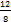=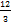Efficiency =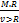x 100%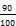=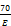/2/3

E= 116.7N